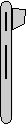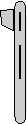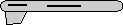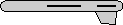# Maths - Euler Angles - Sample Orientations

In order to try to explain things and give some examples we can try I thought it might help to show the rotations for a finite subset of the rotation group. We will use the set of rotations of a cube onto itself, this is a permutation group which gives 24 possible rotations as explaned on this page.

heading applied first giving 4 possible orientations:reference orientation heading = 0 attitude = 0 bank = 0rotate by 90 degrees about y axis heading = 90 degrees attitude = 0 bank = 0rotate by 180 degrees about y axis heading = 180 degrees attitude = 0 bank = 0rotate by 270 degrees about y axis heading = -90 degrees attitude = 0 bank = 0

Then apply attitude +90 degrees for each of the above: (note: that if we went on to apply bank to these it would just rotate between these values, the straight up and streight down orientations are known as singularities because they can be fully defined without using the bank value)heading = 0 attitude = 90 degrees bank = 0heading = 90 degrees attitude = 90 degrees bank = 0heading = 180 degrees attitude = 90 degrees bank = 0heading = -90 degrees attitude = 90 degrees bank = 0

Or instead apply attitude -90 degrees (also a singularity):heading = 0 attitude = -90 degrees bank = 0heading = 90 degrees attitude = -90 degrees bank = 0heading = 180 degrees attitude = -90 degrees bank = 0heading = -90 degrees attitude = -90 degrees bank = 0

Normally we dont go beond attitude + or - 90 degrees because thes are singularities, instead apply bank +90 degrees:heading = 0 attitude = 0 bank = 90 degreesheading = 90 degrees attitude = 0 bank = 90 degreesheading = 180 degrees attitude = 0 bank = 90 degreesheading = -90 degrees attitude = 0 bank = 90 degrees

Apply bank +180 degrees:heading = 0 attitude = 0 bank = 180 degreesheading = 90 degrees attitude = 0 bank = 180 degreesheading = 180 degrees attitude = 0 bank = 180 degreesheading = -90 degrees attitude = 0 bank = 180 degrees

Apply bank -90 degrees:heading = 0 attitude = 0 bank = 90 degreesheading = 90 degrees attitude = 0 bank = 90 degreesheading = 180 degrees attitude = 0 bank = 90 degreesheading = -90 degrees attitude = 0 bank = 90 degrees

encoding of these rotations in quaternions is shown here.
encoding of these rotations in matricies is shown here.
encoding of these rotations in axis-angle is shown here.

The working to convert each of these to matrix is shown here.

 metadata block see also: Correspondence about this page Book Shop - Further reading. Where I can, I have put links to Amazon for books that are relevant to the subject, click on the appropriate country flag to get more details of the book or to buy it from them.3D Math Primer - Aimed at complete beginners to vector and matrix algebra. Other Math Books

This site may have errors. Don't use for critical systems.